Function Repository Resource:

# OrthogonalPolynomialCoefficientConvert

Convert coefficients of a series with respect to one orthogonal polynomial basis into another

Contributed by: Jan Mangaldan
 ResourceFunction["OrthogonalPolynomialCoefficientConvert"][poly,x,basis] gives a list of coefficients ci of the orthogonal polynomial series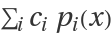that is equal to poly, where pi(x) is represented by the orthogonal polynomial basis. ResourceFunction["OrthogonalPolynomialCoefficientConvert"][cof,basis1→basis2] gives a list of coefficients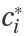of the orthogonal polynomial series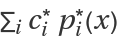that is equal to, where ci is the (i+1)th element of the list cof and pi(x) and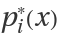are respectively represented by the orthogonal polynomials basis1 and basis2.

## Details

The following polynomial bases are supported:
 "Monomial" monomial basis xi "ChebyshevFirst" Chebyshev polynomial of the first kind ChebyshevT[i,x] "ChebyshevSecond" Chebyshev polynomial of the second kind ChebyshevU[i,x] "Hermite" Hermite polynomial HermiteH[i,x] "Laguerre" Laguerre polynomial LaguerreL[i,x] "Legendre" Legendre polynomial LegendreP[i,x] {"Gegenbauer",m} Gegenbauer polynomial GegenbauerC[i,m,x] {"Laguerre",a} associated Laguerre polynomial LaguerreL[i,a,x] {"Jacobi",a,b} Jacobi polynomial JacobiP[i,a,b,x]
Strings in specifications like "Monomial" and {"Jacobi",a,b} can be replaced with the corresponding symbols, as in Power and {JacobiP,a,b}.

## Examples

### Basic Examples (2)

Get the coefficients of a polynomial in the Legendre basis:

 In:=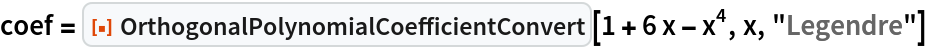Out=Verify that the Legendre coefficients reproduce the original polynomial:

 In:=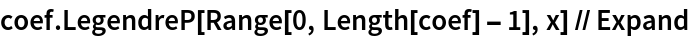Out=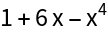Convert coefficients for a Laguerre series to coefficients for a Chebyshev series of the first kind:

 In:=Out=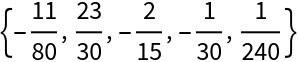Verify the equivalence:

 In:=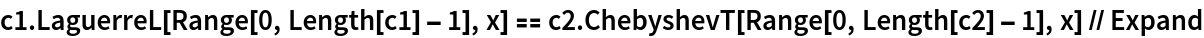Out=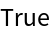### Scope (2)

Convert a Laguerre series with symbolic coefficients and parameters to a Hermite series:

 In:=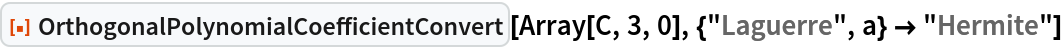Out=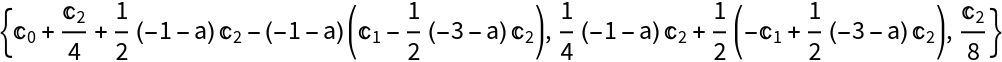An equivalent specification:

 In:=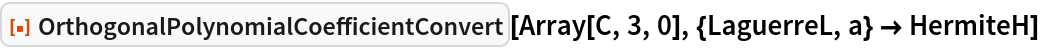Out=Convert monomial basis coefficients to coefficients for a Chebyshev series of the second kind:

 In:=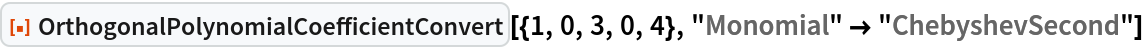Out=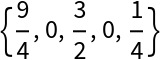An equivalent specification:

 In:=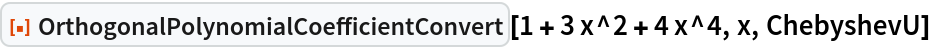Out=### Properties and Relations (2)

Converting an orthogonal polynomial series to the monomial basis is equivalent to expanding the series out and getting its coefficients:

 In:=Out=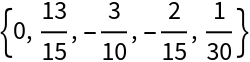In:=Out=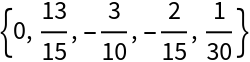OrthogonalPolynomialCoefficientConvert can be used as its own inverse:

 In:=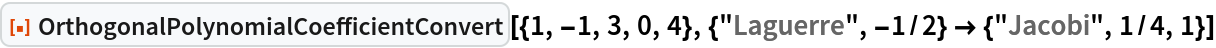Out=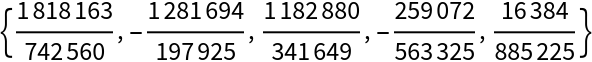In:=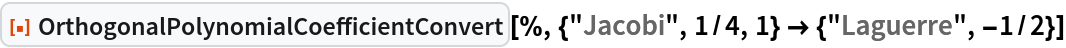Out=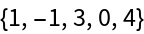### Neat Examples (1)

Express a Jacobi series in terms of another Jacobi series with different parameters:

 In:=Out=## Version History

• 1.0.0 – 05 April 2021